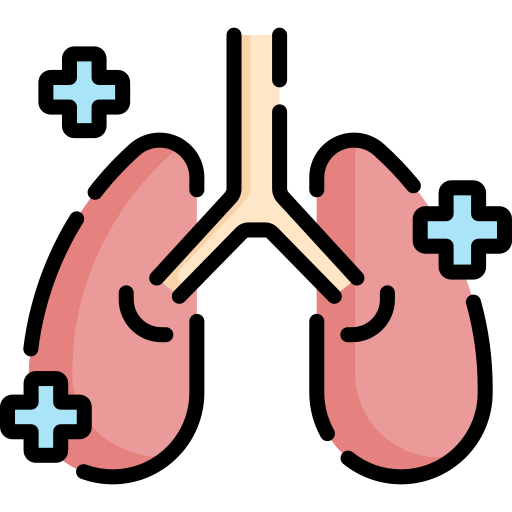# Pulmonary calculators- stepscalculator.com

## You will find answers to your medical questions here, as well as receive a lot of scientifically proven information

•The BODE index score is used to predict the chances of survival of a patient with COPD.
Check BODE index score
•the best tool to monitor patient suffering from community-acquired pneumonia (CAP)
CURB 65 Score Calculator
•Calculate the correct Endotracheal tube size
Endotracheal tube size calculator
•Calculate Light Criteria for Pleural Effusion
Light's Criteria Calculator
•The lung Capacity Calculator helps you determine Total Lung Capacity
Lung Capacity calculator
•The oxygenation Index Calculator finds the PaO₂/FiO₂ ratio
Oxygenation Index Calculator
•Find your pack year for better diagnosis.
Pack year calculator
•This tool is used clinically for asthma patients.
Peak flow Calculator
•Calculate PaO₂/FiO₂ Ratio using this tool.
Online PF Ratio calculator
•online tool that measures and assesses the mortality & risk of Community Acquired Pneumonia
PSI Calculator Online
•Use this online tool to determine the magnitude of cardiac shunt.
QP/QS Ratio Calculator
•an indication of the patient's capability to efficiently breathe on their own.
RSBI calculator-Rapid Shallow Breathing Index.
•Predict the probability of pulmonary embolism(PE)
Revised Geneva Score Calculator
•Predict Ideal ETT depth by entering patient's height
Tidal Volume Calculator
•